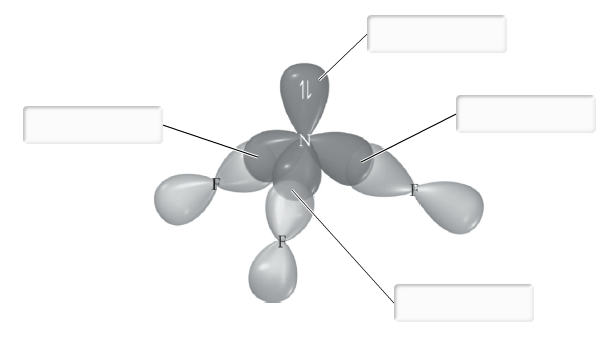# Problem: Label all bonds in NF3.Hybridization of N atom in NF3 is sp3Lone pair in p orbitalLone pair in s orbitalδ: N(p) - F(sp3)δ: N(p) - F(p)π: N(p) - F(p)Lone pair in sp3 orbitalδ: N(sp3) - F(p)π: N(sp3) - F(p)

###### FREE Expert Solution

We are asked to label all the bonds in NF3.

Step 1: Determine the central atom in this molecule.

• N less electronegative atom than F → central atom

Step 2: Calculate the total number of valence electrons present.

NF3:

Group               Valence Electrons

N         5A                   1 × 5 e = 5 e

F          7A                   3 × 7 e = 21 e

Total:  26 valence e

79% (390 ratings)###### Problem Details

Label all bonds in NF3.

Hybridization of N atom in NF3 is sp3Lone pair in p orbital

Lone pair in s orbital

δ: N(p) - F(sp3)

δ: N(p) - F(p)

π: N(p) - F(p)

Lone pair in sp3 orbital

δ: N(sp3) - F(p)

π: N(sp3) - F(p)